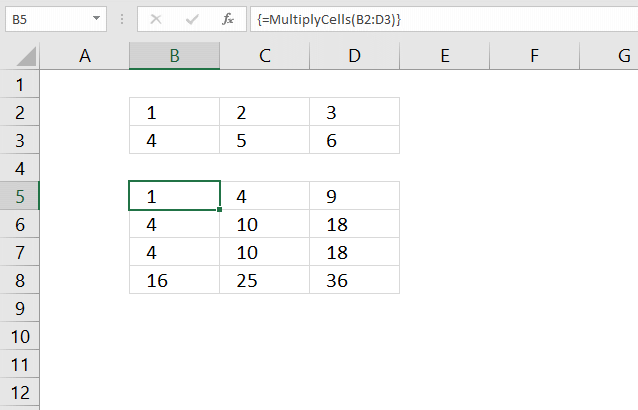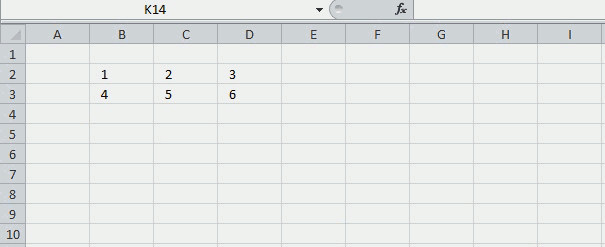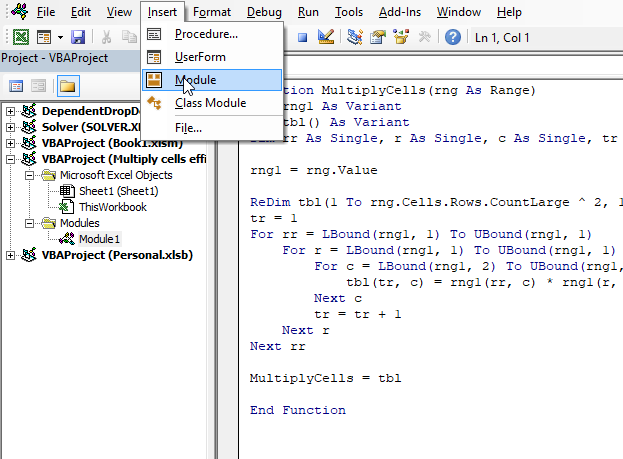Author: Oscar Cronquist Article last updated on February 09, 2023This article demonstrates a User Defined Function (UDF) that multiplies numbers in each row with the remaining rows in a cell range.

The image above demonstrates the UDF in cell range B5:D8, it takes the first row and multiplies the numbers with all rows in the same cell range. It then continues to the second row and multiplies that row with all rows in the cell range.

The total number of rows returned is calculated like this: number_of_rows * number_of_rows. There are two rows in cell range B2:D3 and the UDF returns four rows (2*2 = 4).

Hello Oscar,I have an Excel dataset consisting of 500 rows by 7 columns. I need to generate additional data points from this dataset.I want to multiply (or other function) each row by all 500 rows, creating 250,000 new rows of data.Each cell needs to function as a constant that is multiplied by all the other cells in the same column (which are not acting as constants).How do I do this efficiently? Thanks in advance!

I don't think you can multiply cells like that with an array formula, as far as I know.

Below is a User Defined function I made, it multiplies each row by all other rows in the specified range.

## 1. MultiplyCells - User Defined FunctionThe picture above shows you the udf using values in cell range B2:B3 and returns the calculated values to cell range B5:D8.

This is a UDF (custom function), you need to copy code to the code module before you can use it.

### UDF syntax

=MultiplyCells(range)

### Arguments

There is only one argument in this UDF.

range - Cell range

## 2. VBA Code

```'Name User Defined Function and dimension parameters
Function MultiplyCells(rng As Range)

'Dimension variables and declare data types
Dim rng1 As Variant
Dim tbl() As Variant
Dim rr As Single, r As Single, c As Single, tr As Single

'Save values in range object rng to array variable rng1
rng1 = rng.Value

'Redimension array variable tbl based on rows and columns in range object rng
ReDim tbl(1 To rng.Cells.Rows.CountLarge ^ 2, 1 To rng.Cells.Columns.CountLarge)
tr = 1

'For ... Next statements
For rr = LBound(rng1, 1) To UBound(rng1, 1)
For r = LBound(rng1, 1) To UBound(rng1, 1)
For c = LBound(rng1, 2) To UBound(rng1, 2)

'Multiply values and save the product to array variable tbl
tbl(tr, c) = rng1(rr, c) * rng1(r, c)
Next c

'Add 1 to variable tr and save to variable tr
tr = tr + 1
Next r
Next rr

'Return numbers in array variable tbl to worksheet
MultiplyCells = tbl
End Function

```

## 3. How to enter the UDF as an array formulaEnter this UDF as an array formula, if the range has 2 rows and 3 columns enter the UDF in a cell range with 4 (2*2) rows and 3 columns.

Here are the steps to enter this UDF as an array formula:

1. Select cell range B5:D8.
2. Type the UDF name and argument:
=MultiplyCells(B2:D3)
3. Press and hold CTRL + SHIFT simultaneously.
4. Press Enter once.
5. Release all keys.

If you did it right the formula now has a curly bracket before and after. Like this {=MultiplyCells(B2:D3)}. Don't enter these yourself. If you are unsure, watch the formula bar carefully in the animated picture above.

## 4. Where to put the code?1. Press Alt+ F11 to open the Visual Basic Editor (VBE).
2. Press with mouse on "Insert" on the top menu, see the image above.
3. Press with mouse on "Module" to insert a module to your workbook.
4. Copy VBA code above.
5. Paste to code window, see the image above.

Copy the following code to a module. How to insert a module to a workbook.

Don't forget to save your workbook as a *.xlsm file (macro-enabled workbook) to attach the code.

## 5. Explaining VBA code

The code explained here is located in the code module. You can find the code module in the VB Editor, press Alt+F11 to open the VB Editor. Press with left mouse button on "Module" found in project explorer.

Function name and arguments

A User Defined Function always starts with "Function" and then a name. This UDF has only one argument. Variable rng is a range object, read more about Defining data types.

`Function MultiplyCells(rng As Range)`

Create Custom Functions

Declaring variables

tbl() is an array and a variant variable, tbl has two parentheses meaning it is an array. rng1  is also a variant.  rr, r, c, and tr are Single variables. Read more about Defining data types.

```Dim rng1 As Variant
Dim tbl() As Variant
Dim rr As Single, r As Single, c As Single, tr As Single```

Dim statement

Save values from rng (range object) to rng1 (variant)

This speeds up the function considerably if you are working with large cell ranges. Excel copies all the values from the sheet and puts them in memory (array).

rng1 = rng.Value

Build array

ReDim dimensions the tbl array variant, it has the number of rows of the range argument with the power of 2. The values in the array are numbered from 1 to n. There are as many columns as in the range argument.

ReDimtbl(1 To rng.Cells.Rows.CountLarge ^ 2, 1 Torng.Cells.Columns.CountLarge)

ReDim Statement

Use variables r and c to save values in array

Variable tr keeps track of where to save the next column values in tbl.

tr = 1

For ... Next statement

Repeats a group of statements a specified number of times, here we want to multiply each row by all rows.

```For rr = LBound(rng1, 1) To UBound(rng1, 1)
For r = LBound(rng1, 1) To UBound(rng1, 1)
For c = LBound(rng1, 2) To UBound(rng1, 2)
```

For ... Next statement

Save value to tbl array

The variables tr, c, rr, and r help us keep track of which values to use and where to save.

```tbl(tr, c) = rng1(rr, c) * rng1(r, c)
```

`tr = tr + 1`

Return tbl values to function

`MultiplyCells = tbl`

End udf

A function procedure ends with this statement.

`End Function`